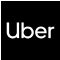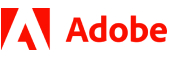New update is available. Click here to update.
Topics

# Redundant Brackets

Easy0/40
Average time to solve is 15m## Problem statement

Given valid mathematical expressions in the form of a string. You are supposed to return true if the given expression contains a pair of redundant brackets, else return false. The given string only contains ‘(‘, ’)’, ‘+’, ‘-’, ‘*’, ‘/’ and lowercase English letters.

Note :

A pair of brackets is said to be redundant when a subexpression is surrounded by needless/ useless brackets.

For Example :
``````((a+b)) has a pair of redundant brackets. The pair of brackets on the first and last index is needless.
While (a + (b*c)) does not have any pair of redundant brackets.
``````
Detailed explanation ( Input/output format, Notes, Images )
Constraints :
``````1 <= T <= 50
3 <= |S| <= 10^4

Time Limit: 1 sec
``````
Sample Input 1 :
``````2
(a+b)
(a+c*b)+(c))
``````
Sample Output 1 :
``````No
Yes
``````
Explanation of Sample Input 1 :
``````In the first test case, there are no redundant brackets. Hence, the output is “No”.

In the second test case, the brackets around the alphabet ‘c’( index 8 and index 10) are redundant. Hence the output is “Yes”.
``````
Sample Input 2 :
``````2
(a*b+(c/d))
((a/b))
``````
Sample Output 2 :
``````No
Yes
``````
Explanation of Sample Input 2 :
``````In the first test case, there are no redundant brackets. Hence, the output is “No”.

In the second test case, the brackets around the subexpression “(a+b)” ( index 0 and index 6) are redundant. Hence the output is “Yes”.
``````Console